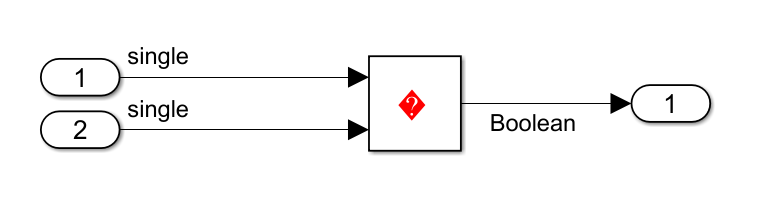Casting modes

Description

Specify how the code generator casts data types for variables.

Category: Code Generation > Code Style

Settings

Default: Nominal

Nominal

Generate code that uses default C compiler data type casting.

For example:

void rtwdemo_rtwecintro_step(void)
{
boolean_T rtb_equal_to_count;
rtDWork.X++;
rtb_equal_to_count = (rtDWork.X != 16);
if (rtb_equal_to_count && (rtPrevZCSigState.Amplifier_Trig_ZCE != POS_ZCSIG))
{ rtY.Output = rtU.Input << 1;
}

Standards Compliant

Generate code where data type casting complies with MISRA® standards.

For example:

void rtwdemo_rtwecintro_step(void)
{
boolean_T rtb_equal_to_count;
rtDWork.X = (uint8_T)((uint32_T)rtDWork.X + 1U);
rtb_equal_to_count = ((int32_T)rtDWork.X != 16);
if (rtb_equal_to_count && ((uint32_T)rtPrevZCSigState.Amplifier_Trig_ZCE !=
POS_ZCSIG)) {
rtY.Output = rtU.Input << 1U;
}

Note

The expression rtY.Output = rtU.Input << 1U is not compliant with MISRA C:12 Rule 10.1 because the model configuration parameter Replace multiplications by powers of two with signed bitwise shift is selected. For more information, see Replace multiplications by powers of two with signed bitwise shifts.

Depending on the setting, the configuration parameter Casting modes can replace bitwise XOR operations with relational operations in the generated code to satisfy the MISRA C:12 Rule 10.1 when the operands are signed types. For example, generate code from the following model with the Casting modes set to Nominal and Standard compliant respectively.// Model step function (casting mode set to Nominal)
void step(void)
{rtY.Out3 = (boolean_T)((int32_T)(rtU.In1 != 0.0F) ^ (int32_T)(rtU.Inport1 !=
0.0F));
}
// Model step function (Casting modes set to Standard Compliant)
void step(void)
{  rtY.Out3 = ((rtU.In1 != 0.0F) != (rtU.Inport1 != 0.0F));
}
Here, the parameters rtU.In1 and rtU.Inport1 are single singed types. Performing a bitwise XOR(^) operation on these operands violates the MISRA C:12 Rule 10.1. To prevent this violation, the code generator replaces the bitwise XOR(^) operation with an inequality(!=) in the generated code when Casting modes is set to Standard compliant.

Explicit

Generate code that casts data type values explicitly.

For example:

void rtwdemo_rtwecintro_step(void)
{
boolean_T rtb_equal_to_count;
rtDWork.X = (uint8_T)((uint32_T)(int32_T)rtDWork.X + 1U);
rtb_equal_to_count = (boolean_T)((int32_T)rtDWork.X != 16);
if (((int32_T)rtb_equal_to_count) && ((int32_T)
rtPrevZCSigState.Amplifier_Trig_ZCE != (int32_T)POS_ZCSIG)) {
rtY.Output = rtU.Input << 1;
}

Command-Line Information

 Parameter: CastingMode Type: character vector Value: 'Nominal' | 'Standards' | 'Explicit' Default: 'Nominal'

Recommended Settings

ApplicationSetting
DebuggingNo impact
TraceabilityNo impact
EfficiencyNo impact
Safety precautionNo impact